# Detailed balance

Now we have to show that this algorithm does satisfy the detailed balance condition: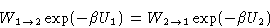(2)
where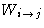is a probability to go from the micro-state i to the micro-state j. In our case the micro-states 1 and 2 differ by the position of one particle, that is moved at a single MC step. The probability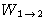is the product of
1.
probability to pick particle 1, and the displacement vector,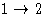, equal to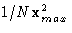, and
2.
probability to accept the move by the Metropolis criterium, equal to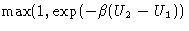The probability of the opposite step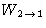is the product of

1.
probability to pick particle 2, and the displacement vector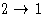, equal to, and
2.
probability to accept the move by the Metropolis criterium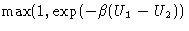If the state 1 has higher energy than 2, then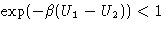, and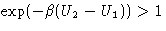, the first contributions are the same in both direct and reverse probabilities, and equation 2 is satisfied.

© 1997 Boris Veytsman and Michael Kotelyanskii
Tue Nov 11 18:36:02 EST 1997